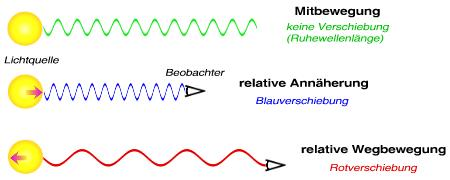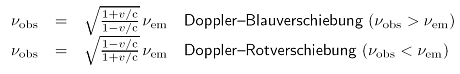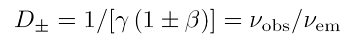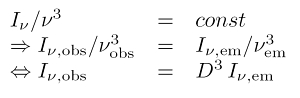# What is the Doppler Effect

## Doppler effect

The Doppler effect is one of the most important phenomena in physics. It is an effect that mathematically describes how the wavelength of a wave changes when the wave exciter or the wave receiver move. We are familiar with this effect in everyday life when the pitch of a sound source moving relative to us changes, e.g. the horn of an ambulance or the noise of the engine in Formula 1. The Doppler effect not only occurs, however with acoustic waves on, but above all with electromagnetic waves - that makes it important for astronomy.

### Namesake

The Doppler effect was named after the Austrian physicist and mathematician Christian Johann Doppler (1803-1853) named. He published the paper in 1842 at the Bohemian Society of Sciences in Prague About the colored light of the double stars and some stars in the sky. In it, Doppler incorrectly modeled light as a longitudinal wave - it had already had in 1821 A.J. Fresnel (1788-1827) claims that light is a transversal Wave is. As we now know, Fresnel was right, but the essence of Doppler's work remains. Doppler also made the remarkable and far-sighted comment that astronomers will use the effect he has discovered to measure the motion and distance of stars - he was proved right.

### acoustic doppler effect

Sound waves propagate in a medium, e.g. air or water, at the speed of sound. The received frequency increases or the received wavelength shortens as the sound source moves towards the receiver. The propagating wave is vividly compressed to a certain extent. If the sound source moves away, the exact opposite happens, because the received frequency becomes lower or the received wavelength is increased. Then, vividly speaking, the propagating wave is stretched.### Doppler effect in light

The Doppler effect of electromagnetic radiation is a little different: light does not need a medium to propagate and can even propagate in a vacuum. For this reason, astronomers receive electromagnetic signals from cosmic sources in the first place. Albert Einstein in the founding of his special theory of relativity in 1905 demanded that light always spreads at the same speed -independently of the state of motion of the light source! This experimentally verified requirement is called Postulate of the constancy of the speed of light in a vacuum c. It has serious consequences for our understanding of space and time. Obviously the Doppler effect of light is completely different from that of acoustic waves, the speed of which can vary.
Phenomenologically, what has been said above is retained: The received light frequency νobs increases or the received wavelength shortens if the light source moves with the relative speed v moved towards the observer. Because this is a change in the light color with the emitted frequency νem towards the blue, physicists call this effect Blueshift.
If the light source moves away, the received radiation frequency becomes smaller or the received light wavelength becomes larger. The physicists then speak of one Redshift, more precisely a Doppler redshift. This is calculated quantitatively with the equations for the special relativistic Doppler effect:### The Doppler factorPhysicists like to write these equations even more compact and use the Lorentz factor γ ist and the normalized speed of light in vacuum β = v / c in the theory of relativity. Rewriting and summarizing the two equations above leads to the equation on the left. In here is D. the Doppler factor. The plus sign is for a Doppler redshift and the minus sign for a Doppler blue shift.
The Doppler factor can be generalized in general relativity (GTR) and is then often no longer called D.but g-factor G, Redshift factor or relativistic generalized Doppler factor (see also A. Müller: The Onset of General Relativity: Gravitationally Redshifted Emission Lines, Astron. Message 327, 1024, 2006; ePrint: astro-ph / 0610791).The Doppler factor or g-factor is of fundamental importance in radiation physics, because on the one hand it is the measure of how theRadiant energy is shifted from the rest system into the observer system and because on the other hand it has a high power Radiation flux, i.e. the measured intensity of the Doppler-shifted radiation. In general, the ratio of the spectral radiation intensity I.ν and third power of the frequency ν - both considered in sameFrame of reference - one Invariant. From this follows the very useful equation on the right to transform from the rest system to the observer system.

### Caution: three types of redshift!

The redshift involves a number of misunderstandings. The Doppler redshift explained above must by no means be allowed to coincide with the cosmological redshift be confused! The background is that the redshift as a shift in the spectrum can have very different physical causes. In the case of the Doppler redshift, the cause is a movement, more precisely a relative movement, which leads to a distance between the emitter and the observer. But there are two other forms of redshift that can only be understood with Einstein's ART. First there is the cosmological redshift. It is based on the fact that a space-time expands. This is exactly what happens with our universe: the whole space (precisely: space-time) expands, so that the light waves contained in it are also pulled apart. This has happened, for example, with the cosmic background radiation since it spread in the recombination epoch (a good 13 billion years ago). Correspondingly, the galaxies are also moving away from each other, because the universe is getting bigger and bigger - it actually happensacceleratedbecause the dark energy is driving the cosmos apart.
It would be now totally wrong to use cosmological redshifts to calculate cosmic escape velocities of distant galaxies. That this does not make sense can be quickly recognized by the fact that, for systems that are far away, this escape speed exceeds the vacuum speed of light that cannot be exceeded according to Einstein. Such calculations make no sense, especially since a concept of speed for an expanding spacetime lattice would be of dubious value.
The final form of redshift is that Gravitational redshift. It can only be understood with Einstein's ART. This is because light loses energy when it spreads in a gravitational field. In Einstein's language, gravity is one curved spacetime and light follows excellent orbits here, the so-called zero geodesics. Vividly argued, the light waves fight like a rocket taking off with gravity and lose energy. While a rocket runs out of fuel, the loss of energy for photons means a shift towards the red. In this case the physicist speaks of a gravitational redshift because the cause of the redshift is gravitation. This effect becomes extreme with a black hole: at the event horizon the light waves have lost all their energy and intensity to gravity. This gives the hole its characteristic blackness.
In general, an observed redshift is the sum of these three redshifts, i.e. Doppler redshift, gravitational redshift and cosmological redshift. This of course complicates the astronomer's work, and he needs more information about the emitter in order to find out the weighting of the redshifts.